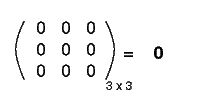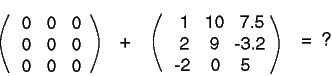What 3x2 matrix could be added to a second 3x2 matrix without changing that second matrix?

The 3x2 matrix that has all its elements zero.

# Zero Matrix

A zero matrix is one which has all its elements zero. Here is a 3x3 zero matrix:The name of a zero matrix is a bold-face zero: 0, although sometimes people forget to make it bold face. Here is an interesting problem:### QUESTION 8:

Form the above sum. No electronic calculators allowed!